Precalculus : Solve Trigonometric Equations and Inequalities in Quadratic Form

Example Questions

Example Question #1 : Solve Trigonometric Equations And Inequalities In Quadratic Form

If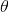exists in the domain from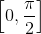, solve the following: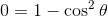Explanation:

Factorize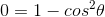.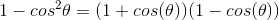Set both terms equal to zero and solve.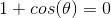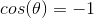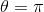This value is not within the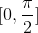domain.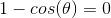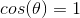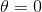This is the only correct value in thedomain.

Example Question #1 : Solving Trigonometric Equations And Inequalities

Solve forin the equation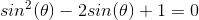on the interval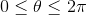.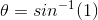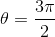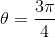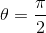Explanation:

If you substitute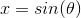you obtain a recognizable quadratic equation which can be solved for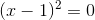.

Then we can plugback into our equation and use the unit circle to find that.

Example Question #1 : Solving Trigonometric Equations And Inequalities

Given that theta exists from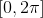, solve: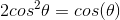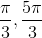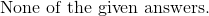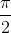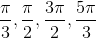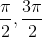Explanation:

In order to solveappropriately, do not divide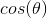on both sides.  The effect will eliminate one of the roots of this trig function.

Substractfrom both sides.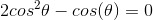Factor the left side of the equation.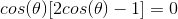Set each term equal to zero, and solve for theta with the restriction.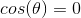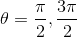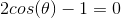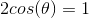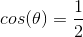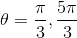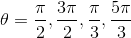Example Question #4 : Solve Trigonometric Equations And Inequalities In Quadratic Form

Solve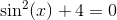for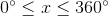There is no solution.There is no solution.

Explanation:

By subtractingfrom both sides of the original equation, we get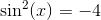. We know that the square of a trigonometric identity cannot be negative, regardless of the input, so there can be no solution.

Example Question #5 : Solve Trigonometric Equations And Inequalities In Quadratic Form

Solve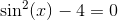whenThere are no solutions.There are no solutions.

Explanation:

Given that, for any input,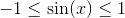, we know that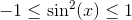, and so the equation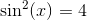can have no solutions.

Example Question #1 : Solve Trigonometric Equations And Inequalities In Quadratic Form

Solve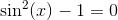whenThere are no solutions.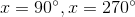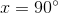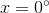By adding one to both sides of the original equation, we get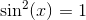, and by taking the square root of both sides of this, we get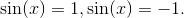From there, we get that, on the given interval, the only solutions areand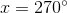.